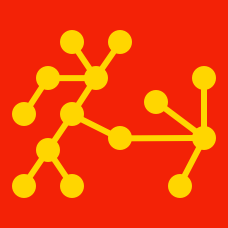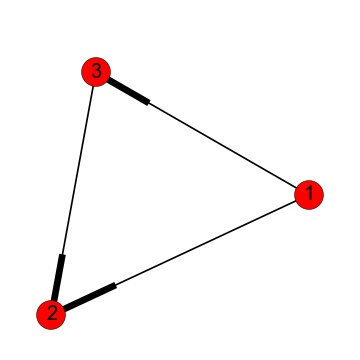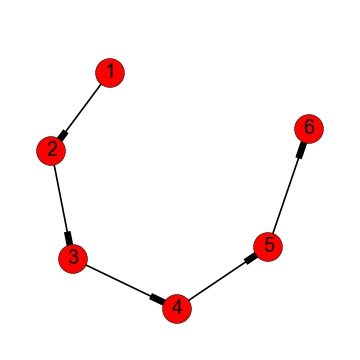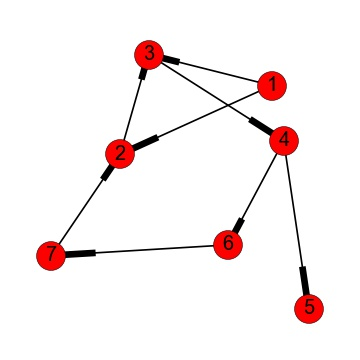Probability

# Directed GraphsIs there a valid path on this directed graph from node 3 to node 1?

Note: the edges are directed toward their arrowhead (depicted as the thicker end). For example, the edge between nodes 2 and 3 is an edge from node 3 to node 2. It can only be traversed in that direction.How many paths of length 2 are there on this directed graph?

Note: the thicker end of an edge is an arrow head. For instance, the edge between nodes 1 and 2 signifies an edge from node 1 to node 2 (where the order is important).

Note: the length of a path is the number of edges in it.

How many distinct (undirected) graphs are there with nodes labeled 1, 2, 3, 4?

How many distinct directed graphs are there with nodes labeled 1, 2, 3, 4, 5?

Clarification: for the purpose of this question, we consider it impossible for there to be bi-directional edges. That is, for any two nodes $A$ and $B$, there is either no edge between them, there is an edge from $A$ to $B$, or there is an edge from $B$ to $A$ (but never both an edge from $A$ to $B$ and one from $B$ to $A$).Is there a path from node 3 to node 2 on the directed graph above?

×